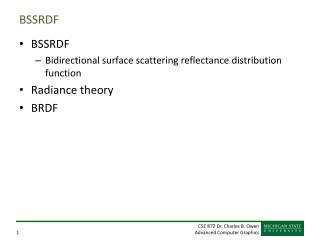DownloadDownload PresentationBSSRDF

# BSSRDF

Télécharger la présentation## BSSRDF

- - - - - - - - - - - - - - - - - - - - - - - - - - - E N D - - - - - - - - - - - - - - - - - - - - - - - - - - -
##### Presentation Transcript

1. BSSRDF • BSSRDF • Bidirectional surface scattering reflectance distribution function • Radiance theory • BRDF

2. Subsurface scattering • Lighting approximations based on only surface reflection fails for: • Translucent materials • Marble, cloth, paper, skin, milk, cheese, bread, meat, fruits, plants, fish, water, snow, etc. • Heck, darn near everything

3. What is subsurface transport? Skin Flesh Bone

4. Radiance Theory • Outgoing radiance equation BSSRDF x0 – Surface point we are computing forw0 – View direction for point x0Fi(xi,wi) – Incident flux on point xi from direction wiFlux = rate of energy per unit time. If xi=x0, we get BRDF – Bidirectional reflectance distribution function (surface only!)

5. Solving for radiance x0 – Surface point we are computing forw0 – View direction for point x0Li(xi,wi) – Incident flux on point xi from direction wiFlux = rate of energy per unit time. Okay, how do we solve for this, assuming we have an equation for S?

6. Simplifying assumption • We’ll only model first order events • Single reflections • We’ll cheat and add a “term” to simulate all other events • “Each scattering event blurs the light distribution, and as a result the light distribution tends toward uniformity as the number of scattering events increases”

7. The equation That’s all there is to it, we can all go home now…

8. The equation Diffusion term Single scattering term

9. Diffusion approximation

10. Strange new worlds • Light hitting a surface and diffusing below the surface is simulated with two light sources • A positive (real) light source below the surface • A negative (virtual) light source above the surface D = Diffusion constant

11. Parameters for this • Effective transport coefficient • Absorption coefficient (material property) • Reduced scattering coefficient (material property)

12. Big ugly equation Albedo Fresnel transmittance

13. Single scattering

14. Single Scattering Term Exercise for the viewer: Determine what S(1) is from the above equations. Note the change of variable. (ouch) Phase function – Distribution that describes the scattering of light to a given angle. Combined extinction coefficient – How much loss as we pass through the material.

15. Obtaining parameters

16. Doing this in a Monte Carlo ray tracer • For each ray • Integrate over random points around the ray intersection to compute diffusion term • Integrate over random distances into the material to compute the single scattering term How do we get the areas?

17. Simple optimizations • Falloff is exponential with distance for both terms • What does that give us? • Is anything redundant happening here?

18. Caveat Emptor • The dipole approximation assumes a flat surface • Assumes only one surface layer Be these problems?